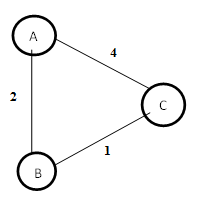# Distance Vector Routing (DVR) Protocol

In distance-vector routing (DVR), each router is required to inform the topology changes to its neighboring routers periodically. Historically it is known as the old ARPNET routing algorithm or Bellman-Ford algorithm.

## How the DVR Protocol Works

• In DVR, each router maintains a routing table. It contains only one entry for each router. It contains two parts − a preferred outgoing line to use for that destination and an estimate of time (delay). Tables are updated by exchanging the information with the neighbor’s nodes.
• Each router knows the delay in reaching its neighbors (Ex − send echo request).
• Routers periodically exchange routing tables with each of their neighbors.
• It compares the delay in its local table with the delay in the neighbor’s table and the cost of reaching that neighbor.
• If the path via the neighbor has a lower cost, then the router updates its local table to forward packets to the neighbor.

## Example − Distance Vector Router Protocol

In the network shown below, there are three routers, A, B, and C, with the following weights − AB =2, BC =3 and CA =5.

Step 1 − In this DVR network, each router shares its routing table with every neighbor. For example, A will share its routing table with neighbors B and C and neighbors B and C will share their routing table with A.Form A A B C A 0 2 3 B C

 Form B A B C A B 2 0 1 C

 Form C A B C A B C 3 1 0

Step 2 − If the path via a neighbor has a lower cost, then the router updates its local table to forward packets to the neighbor. In this table, the router updates the lower cost for A and C by updating the new weight from 4 to 3 in router A and from 4 to 3 in router C.

 Form A A B C A 0 2 3 B C

 Form B A B C A B 2 0 1 C

 Form C A B C A B C 3 1 0

Step 3 − The final updated routing table with lower cost distance vector routing protocol for all routers A, B, and C is given below −

### Router A

 Form A A B C A 0 2 3 B 2 0 1 C 3 1 0

### Router B

 Form B A B C A 0 2 3 B 2 0 1 C 3 1 0

### Router C

 Form C A B C A 0 2 3 B 2 0 1 C 3 1 0